# ACT Math: Linear Equations Chapter Exam

Exam Instructions:

Choose your answers to the questions and click 'Next' to see the next set of questions. You can skip questions if you would like and come back to them later with the yellow "Go To First Skipped Question" button. When you have completed the practice exam, a green submit button will appear. Click it to see your results. Good luck!

### Page 1

#### Question 1 1. Which of these equations represents a line that is perpendicular to the line in the graph below?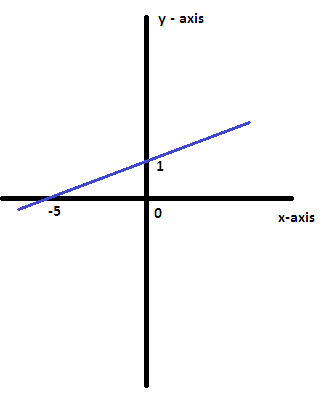### Page 2

#### Question 7 7. Based on this graph, what is the solution to the system of equations?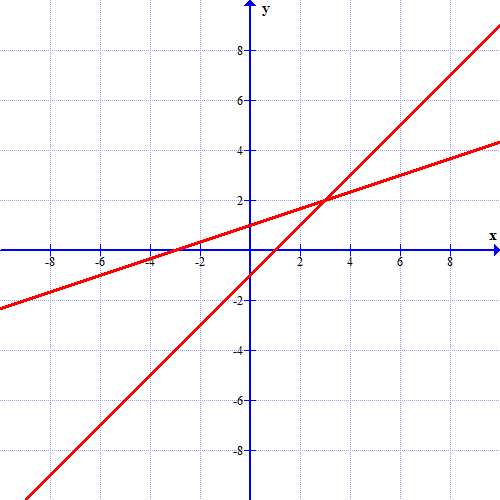### Page 3

#### Question 11 11. Which of the following is a solution to the system of equations shown in the graph below?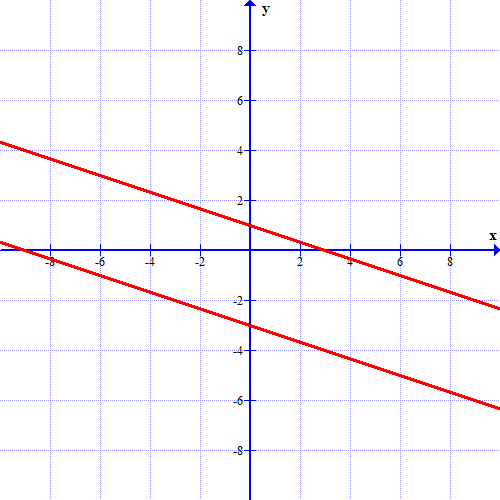#### Question 15 15. Which linear equation is shown below?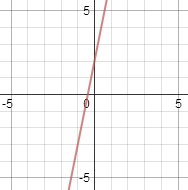### Page 5

#### Question 21 21. What is the equation of the graph below?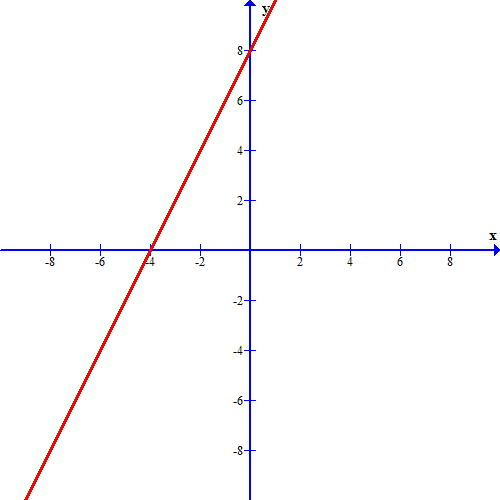#### ACT Math: Linear Equations Chapter Exam Instructions

Choose your answers to the questions and click 'Next' to see the next set of questions. You can skip questions if you would like and come back to them later with the yellow "Go To First Skipped Question" button. When you have completed the practice exam, a green submit button will appear. Click it to see your results. Good luck!

Support